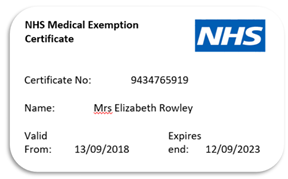Dec 23
 NHS (UK) Number Identity

Everyone registered with the National Health Service (NHS) in England, Wales and the Isle of Man has a unique patient identifier called an NHS Number. The modern style of NHS number was generally introduced in 1996 and one allocated to every newborn since July 1995 and becoming mandatory on 1 April 1997.The NHS Number helps healthcare staff and service providers identify you correctly and match your details to your health records. The number will appear on most official documents.

Each NHS Number consists of up to 10 digits shown in a 3-3-4 format. Those having NHS numbers following in the format XXXX 999 are no longer valid.

## The Validation

In the NHS example above "9434765919" is used for the following below validation.

• The first digit is 9. This is multiplied by 10.
• The second digit is 4. This is multiplied by 9.
• And so on until the ninth digit (1) is multiplied by 2.
• The result of this calculation is summed. In this example:
• 9*10+4*9+3*8+4*7+7*6+6*5+5*4+9*3+1*2 = 299.
• The remainder when dividing this number by 11 is calculated, yielding a number in the range 0–10, which would be 2 in this case.
• Finally, this number is subtracted from 11 to give the checksum in the range 1–11, in this case 9, which becomes the last digit of the NHS number.
• A checksum of 11 is represented by 0 in the final NHS number. If the checksum is 10 then the number is not valid.